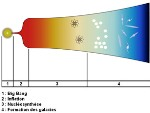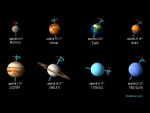# Horizon

## Distance of the horizonAutomatic translation Category: Earth
Updated June 01, 2013

Where is the horizon?
For a man of 1.80 meters located at the edge of the sea, the horizon is approximately 4789 meters, only.

How to calculate the distance to the horizon?
Next figure at right, we will take as the distance of the radius of the Earth the mean radius 6 371 km.

R = radius of Earth = 6371000 m
h = height of the observation = 1.8 m
D'= distance of the horizon

D2 + R2 = (R + h)2
D2 + R2 = R2 + 2hR + h2
D2 = 2hR + h2
D = √ (2hR + h2)

Let D = 4 789.11 meters

nota: Equatorial radius of Earth = 6 378.137 km
Polar radius of Earth = 6 356.752 km
Mean radius = 6 371.0 kilometers

 Height of the observer Distance of the horizon 1 m 3 569 m 1.80 m 4 789 m 5 m 7 981 m 10 m 11 288 m 20 m 15 963 m 50 m 25 240 m 100 m 35 696 m 500 m 79 820 m 1 km 112 884 m 5 km 252 457 m 10 km 357 099 m 20 km 505 212 m 50 km 799 749 m 100 km 1 133 225 m 200 km 1 608 850 m 500 km 2 573 130 m## Horizon and curvature of the Earth

The horizon on Earth, is the line that limits the distant view. This line seems to separate the sky from the earth or the sea. The circular line of the horizon does not lie at an infinite distance to the contrary, it is quite close but the distance varies depending on the height of the observation. The observation line of the horizon is tangent to the surface and limits what can be observed because of his own position or situation. The horizon takes into account the curvature of the Earth.

nota: In astronomy, the cosmic horizon or cosmological horizon means the limit of the observable Universe from Earth.

### Image: On this beautiful sunset far, you can see the curvature of the Earth's atmosphere. This image of the horizon of the Earth was taken by the crew of STS-129 Space Shuttle international. Image Credit: NASA1997 © Astronoo.com − Astronomy, Astrophysics, Evolution and Earth science.Big Bang mystery,horizon problem...Earthin an aquarium...Axis of rotation ofplanets or obliquity...Zodiacal light...The rainbow and colors...